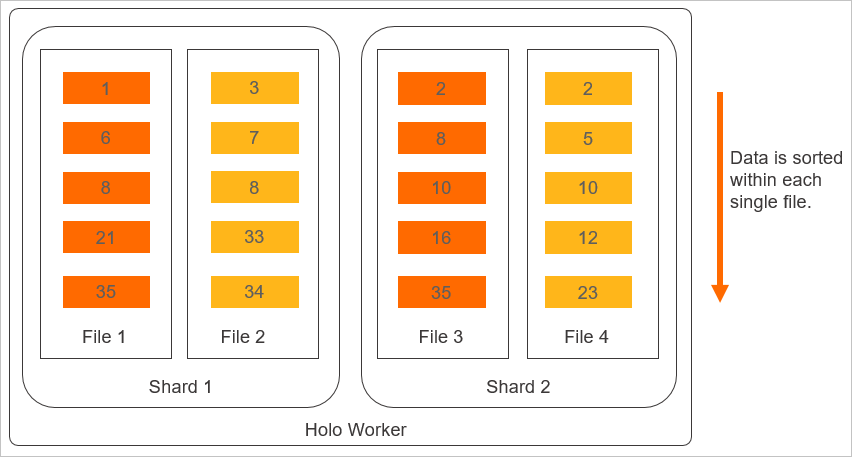This topic introduces the concept of clustering key in Hologres and describes how to use a clustering key to accelerate queries.

## Overview

A clustering key determines the layout of clusters in a file, which means that the data in the file is sorted based on the clustering key. A clustering key in Hologres is different from a clustered index in a MySQL or SQL Server database. A clustered index is used to sort the data of a whole table. A clustering key in Hologres is used to sort only data in a specific file, and therefore is less cost-effective. You can set a clustering key for a table only once. If you change the clustering key, you must import data to the table again.
• For a row-oriented table with a primary key, the primary key is the default clustering key. If a row-oriented table has no primary key, no default clustering key is set.
• No default clustering key is set for a column-oriented table.

## Logical layout of query results based on a clustering key

Clustering key-based queries follow the leftmost matching principle. Query results that do not match a clustering key based on this principle cannot be sorted by the clustering key. The following example shows the logical layout of query results based on a clustering key in Hologres.

Create a table that contains three fields: Name, Date, and Class. If you set different fields as the clustering key, the sorting results are different, as shown in the following figure.• If you set the Date field as the clustering key, the data in the table is sorted based on the values of the Date field.
• If you set the Class and Date fields as the clustering key, the data in the table is sorted first based on the values of the Class field and then based on the values of the Date field. Clustering key-based queries follow the leftmost matching principle. Therefore, if a clustering key consists of three fields a, b, and c, data can be sorted based on the matching of the a and b fields instead of the b and c fields.

## Layout of physical storage based on a clustering key

The following figure shows the layout of physical storage based on a clustering key.## Scenarios

A clustering key is suitable for point queries and range queries. It can optimize the performance of filter operations. For example, a clustering key can accelerate a query that contains the condition `where a = 1` or `where a > 1 and a < 5`. You can set a field as the clustering key and create a bitmap index for the field at the same time. This provides optimum point query performance.

A Hologres file consists of blocks. For a query, a clustering key is used to find the block where the queried data resides. A bitmap index is used to find the queried data in the block. Therefore, you can use a clustering key together with bitmap indexes to achieve optimum performance of file data filtering. The following examples show the similarity and differences between a clustering key and a bitmap index.
• Similarity: Both a clustering key and a bitmap index can accelerate point queries. The following statement is a sample point query:
``select a,b,c from test_b where b = 10;``
• Differences: You can set only one clustering key for each table. A clustering key can accelerate range queries. You can set multiple bitmap indexes for each table. Bitmap indexes cannot accelerate range queries. If you need to perform different point queries, you can set the most frequently queried field as the clustering key and create bitmap indexes for less frequently queried fields. The following sample statements show the details:
``````select a,b,c from test_b where a = 10;
select a,b,c from test_b where a = 20;
select a,b,c from test_b where b = 20;
select a,b,c from test_b where c = 20;
--Set the a field as the clustering key and create bitmap indexes for the b and c fields.
--call set_table_property('test_b', 'clustering_key', 'a');
--call set_table_property('test_b', 'bitmap_columns', 'a,b,c');``````

## Examples

You can use a clustering key together with bitmap indexes to achieve optimum performance of file data filtering, as shown in the following examples:
``````select a,b,c from test_a where a > 10 and a < 100;
--Set the a field as the clustering key.
--call set_table_property('test_a', 'clustering_key', 'a');

select a,b,c from test_b where b = 10;
--Set the b field as the clustering key and create a bitmap index for the b field.
--call set_table_property('test_b', 'clustering_key', 'b');
--call set_table_property('test_b', 'bitmap_columns', 'b');``````